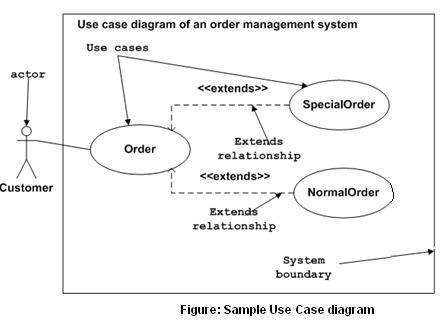# UML - Use Case Diagrams

To model a system, the most important aspect is to capture the dynamic behavior. Dynamic behavior means the behavior of the system when it is running/operating.

Only static behavior is not sufficient to model a system rather dynamic behavior is more important than static behavior. In UML, there are five diagrams available to model the dynamic nature and use case diagram is one of them. Now as we have to discuss that the use case diagram is dynamic in nature, there should be some internal or external factors for making the interaction.

These internal and external agents are known as actors. Use case diagrams consists of actors, use cases and their relationships. The diagram is used to model the system/subsystem of an application. A single use case diagram captures a particular functionality of a system.

Hence to model the entire system, a number of use case diagrams are used.

## Purpose of Use Case Diagrams

The purpose of use case diagram is to capture the dynamic aspect of a system. However, this definition is too generic to describe the purpose, as other four diagrams (activity, sequence, collaboration, and Statechart) also have the same purpose. We will look into some specific purpose, which will distinguish it from other four diagrams.

Use case diagrams are used to gather the requirements of a system including internal and external influences. These requirements are mostly design requirements. Hence, when a system is analyzed to gather its functionalities, use cases are prepared and actors are identified.

When the initial task is complete, use case diagrams are modelled to present the outside view.

In brief, the purposes of use case diagrams can be said to be as follows −

• Used to gather the requirements of a system.

• Used to get an outside view of a system.

• Identify the external and internal factors influencing the system.

• Show the interaction among the requirements are actors.

## How to Draw a Use Case Diagram?

Use case diagrams are considered for high level requirement analysis of a system. When the requirements of a system are analyzed, the functionalities are captured in use cases.

We can say that use cases are nothing but the system functionalities written in an organized manner. The second thing which is relevant to use cases are the actors. Actors can be defined as something that interacts with the system.

Actors can be a human user, some internal applications, or may be some external applications. When we are planning to draw a use case diagram, we should have the following items identified.

• Functionalities to be represented as use case

• Actors

• Relationships among the use cases and actors.

Use case diagrams are drawn to capture the functional requirements of a system. After identifying the above items, we have to use the following guidelines to draw an efficient use case diagram

• The name of a use case is very important. The name should be chosen in such a way so that it can identify the functionalities performed.

• Give a suitable name for actors.

• Show relationships and dependencies clearly in the diagram.

• Do not try to include all types of relationships, as the main purpose of the diagram is to identify the requirements.

• Use notes whenever required to clarify some important points.

Following is a sample use case diagram representing the order management system. Hence, if we look into the diagram then we will find three use cases (Order, SpecialOrder, and NormalOrder) and one actor which is the customer.

The SpecialOrder and NormalOrder use cases are extended from Order use case. Hence, they have extended relationship. Another important point is to identify the system boundary, which is shown in the picture. The actor Customer lies outside the system as it is an external user of the system.## Where to Use a Use Case Diagram?

As we have already discussed there are five diagrams in UML to model the dynamic view of a system. Now each and every model has some specific purpose to use. Actually these specific purposes are different angles of a running system.

To understand the dynamics of a system, we need to use different types of diagrams. Use case diagram is one of them and its specific purpose is to gather system requirements and actors.

Use case diagrams specify the events of a system and their flows. But use case diagram never describes how they are implemented. Use case diagram can be imagined as a black box where only the input, output, and the function of the black box is known.

These diagrams are used at a very high level of design. This high level design is refined again and again to get a complete and practical picture of the system. A well-structured use case also describes the pre-condition, post condition, and exceptions. These extra elements are used to make test cases when performing the testing.

Although use case is not a good candidate for forward and reverse engineering, still they are used in a slightly different way to make forward and reverse engineering. The same is true for reverse engineering. Use case diagram is used differently to make it suitable for reverse engineering.

In forward engineering, use case diagrams are used to make test cases and in reverse engineering use cases are used to prepare the requirement details from the existing application.

Use case diagrams can be used for −

• Requirement analysis and high level design.

• Model the context of a system.

• Reverse engineering.

• Forward engineering.